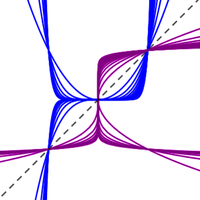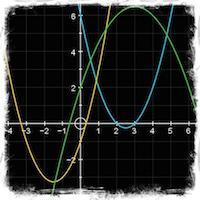# Functions

##Relationships, functions and modelling!

This section is all about exploring the relationship between two variables. How does one change as the other does? Is the price of a service directly proportional to the length of time you use it? Do temperature variations where you live follow the same pattern every year? How is the world population growing? How are profits growing in your company? How do expect that to change over time? The whole principle about mathematical modelling is to explore how one tings changes with respect to another and to try and deduce a mathematical function that describes it so that we might use that to explain what is happening and predict what might happen in the future. It is a complex field and far reaching field of mathematics that is increasingly topical and useful. Here you can learn about some of the key models that we use!

## What is in this section?

#### 2.1 & 2.2 Introducing Functions

Functions are extraordinary algebraic and visual tools which allow us to understand and analyse relationships. In this section you will learn exactly what a function is and how to find a function's inverse, alongside developing a more nuanced...

#### 2.3 & 2.4 Key Features of Functions And Graphing

Now that we know something about the basics of functions, this section aims to help deepen your understanding of them and enable you to identify key features such as vertex points, intercepts with axes and asymptotes. You will also learn about...

#### 2.5 Linear models

Commonly seen in the form y = f(x) = mx + c where m represents the gradient/rate of change of the function and c is the y-intercept, where x = 0. These models are a fundamental bit of algebra that come up again and again. they are also fairly...

Quadratic functions are in the form ax2 + bx + c, and have fascinated mathematicians for centuries. They have some fundamental properties that will explored in this unit. Probably the best known example of quadratic models are for projectiles....

#### 2.5 Exponential Models

The exponential function is set apart from other families of functions because of the fundamental impact of having the variable in the exponent. It stops being a multiplier and starts being more 'powerful' (forgive the bad joke). These functions...

#### 2.5 Trigonometric Models

The Mathematics of Periodic Phenomena Phenomena which display periodic nature, such as the motion of tides, the orbits of planets, or the spinning of a ferris wheel, that we can understand mathematically using trigonometic models. Once we develop...

## Key Questions

The following are list of key questions that you might be asking about these topics with links to the places where you can get the answers!!!

## What is a linear function?

Linear functions are those which graph a straight line. Learn how to work with them here:  2.5 Linear models

## What is a function?

Functions have specific properties which distinguish them from relations.  2.1 & 2.2 Introducing Functions

## What is some key features of functions?

Functions have specific features such as  stationary points, asymptotes and intercepts that we need to bear in mind. In an exam, you may also be asked to draw or sketch them.  An introduction to the key features of functions and graphing is here.  2.3 & 2.4 Key Features of Functions And Graphing

## What are the features of quadratic models?

Quadratic graphs and equations have many interesting features, such as turning points and symmetry which make them very useful to solve real life problems.  2.5 Quadratic Models

## What are the key features of trigonometric models?

Trigonometric models are used to model all sorts of real life situations such as tides, length of days, and even ferris wheels.  Find out about trigonometric models.  2.5 Trigonometric Models

## What are exponential models for?

Exponential models are used to model many types of natural phenomena, such as population, spread of disease, and interest rates.  2.5 Exponential Models

•#### 2.1 & 2.2 Introducing Functions

Functions are extraordinary algebraic and visual tools which allow us to understand and analyse relationships. In this section you will learn exactly what a function is and how to find a function's inverse, alongside developing a more nuanced...

•#### 2.3 & 2.4 Key Features of Functions And Graphing

Now that we know something about the basics of functions, this section aims to help deepen your understanding of them and enable you to identify key features such as vertex points, intercepts with axes and asymptotes. You will also learn about...

•#### 2.5 Linear models

Commonly seen in the form y = f(x) = mx + c where m represents the gradient/rate of change of the function and c is the y-intercept, where x = 0. These models are a fundamental bit of algebra that come up again and again. they are also fairly...

•Quadratic functions are in the form ax2 + bx + c, and have fascinated mathematicians for centuries. They have some fundamental properties that will explored in this unit. Probably the best known example of quadratic models are for projectiles....

•#### 2.5 Exponential Models

The exponential function is set apart from other families of functions because of the fundamental impact of having the variable in the exponent. It stops being a multiplier and starts being more 'powerful' (forgive the bad joke). These functions...

•#### 2.5 Trigonometric Models

The Mathematics of Periodic Phenomena Phenomena which display periodic nature, such as the motion of tides, the orbits of planets, or the spinning of a ferris wheel, that we can understand mathematically using trigonometic models. Once we develop...